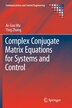# Complex Conjugate Matrix Equations For Systems And Control

## byAi-guo Wu, Ying Zhang

### Pricing and Purchase Info

\$190.00 online
\$193.50 list price
Earn 950 plum® points

Prices and offers may vary in store

Quantity:

In stock online

Ships free on orders over \$25

Not available in stores

The book is the first book on complex matrix equations including the conjugate of unknown matrices. The study of these conjugate matrix equations is motivated by the investigations on stabilization and model reference tracking control for discrete-time antilinear systems, which are a particular kind of complex system with structure constraints. It proposes useful approaches to obtain iterative solutions or explicit solutions for several types of complex conjugate matrix equation. It observes that there are some significant differences between the real/complex matrix equations and the complex conjugate matrix equations. For example, the solvability of a real Sylvester matrix equation can be characterized by matrix similarity; however, the solvability of the con-Sylvester matrix equation in complex conjugate form is related to the concept of con-similarity. In addition, the new concept of conjugate product for complex polynomial matrices is also proposed in order to establish a unified approach for solving a type of complex matrix equation.
Title:Complex Conjugate Matrix Equations For Systems And ControlFormat:PaperbackDimensions:487 pages, 9.41 × 7.24 × 0.98 inPublished:June 12, 2018Publisher:Springer NatureLanguage:English

The following ISBNs are associated with this title:

ISBN - 10:9811092168

ISBN - 13:9789811092169